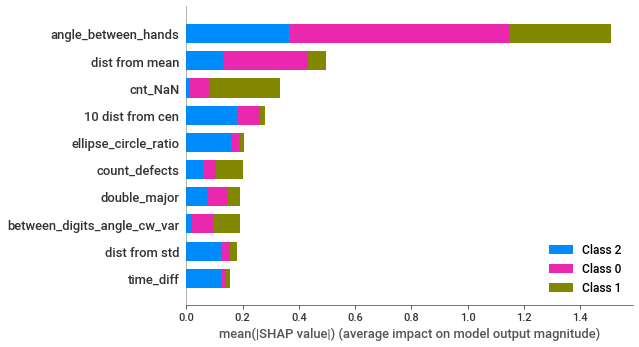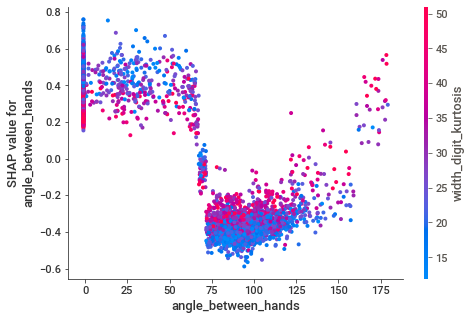# F1:0.52-Baseline Imbalance Samplers(20+) and 8Classifiers

Automated Benchmark of Imbalanced Samplers and Classifiers + Feature Engineering with Shapley Values

This notebook gets a score of 0.521 F1 Score and log loss of 0.669.

The notebook was built upon the features shared in the link - https://discourse.aicrowd.com/t/target-distribution-in-the-test-set-lb-0-616-with-a-simple-magic-trick/5613

Created new features of mean/std-based features and checked for importance using Shapley values (https://shap.readthedocs.io/en/latest/index.html) and check for the impact of features on the normal diagnosis probability. The feature dist from mean and dist from std created by averaging and taking standard deviation across the digits for  dist from cen  feature showed higher importance based on Shapley values.

About 20+ samples and 8 classifier models (including the popular Xgboost, LightGBM, Catboost, and Tensorflow based Keras Neural Network Classifier) were used for the benchmarking. Random Forest tends to give the best cv scores but Catboost does better on the leaderboard.

This selects the best model based on the K-Fold metric. Alternatively, a stratified k-fold metric can also be chosen. Any other strategy like a train -valid split can also be easily added by including it in the list of model_sel_strategy. A simple K-fold was selected by checking proximity to leaderboard scores.

The scikit learn and imbalanced Learn pipelines have been used to automate the benchmarking process over all the samplers and classifiers.

Standard parameters for the classifier and samplers were used without hyper parameter tuning which could further boost performance.  Log loss score was high as some of the probabilities were quite spread across the classes. A simple ensemble-based approach of arithmetic/geometric mean or just averaging based on different models selected in different k-folds could help to gain more confidence in the probabilities.# What is the notebook about?¶

The challenge is to use the features extracted from the Clock Drawing Test to build an automated and algorithm to predict whether each participant is one of three phases:

1) Pre-Alzheimer’s (Early Warning) 2) Post-Alzheimer’s (Detection) 3) Normal (Not an Alzheimer’s patient)

In machine learning terms: this is a 3-class classification task.

# How to use this notebook? 📝¶• Update the config parameters. You can define the common variables here
Variable Description
AICROWD_DATASET_PATH Path to the file containing test data (The data will be available at /ds_shared_drive/ on aridhia workspace). This should be an absolute path.
AICROWD_PREDICTIONS_PATH Path to write the output to.
AICROWD_ASSETS_DIR In case your notebook needs additional files (like model weights, etc.,), you can add them to a directory and specify the path to the directory here (please specify relative path). The contents of this directory will be sent to AIcrowd for evaluation.
AICROWD_API_KEY In order to submit your code to AIcrowd, you need to provide your account's API key. This key is available at https://www.aicrowd.com/participants/me
• Installing packages. Please use the Install packages 🗃 section to install the packages
• Training your models. All the code within the Training phase ⚙️ section will be skipped during evaluation. Please make sure to save your model weights in the assets directory and load them in the predictions phase section

# Setup AIcrowd Utilities 🛠¶

We use this to bundle the files for submission and create a submission on AIcrowd. Do not edit this block.

In :
!pip install -q -U aicrowd-cli

In :
%load_ext aicrowd.magic

In :
!pip install sweetviz
!pip install -U jupyter

In :
import sweetviz as sv

In :
import os

# Please use the absolute for the location of the pip install Shapelydataset.
# Or you can use relative path with os.getcwd() + "test_data/validation.csv"
AICROWD_DATASET_PATH = os.getenv("DATASET_PATH", "/ds_shared_drive/validation.csv")
AICROWD_PREDICTIONS_PATH = os.getenv("PREDICTIONS_PATH", "predictions.csv")
AICROWD_ASSETS_DIR = "assets"

In :
#!pip install ipywidgets
#!jupyter nbextension enable --py widgetsnbextension
#!conda install -y jupyterlab_widgets


# Install packages 🗃¶

In :
!pip install numpy pandas
!pip install -U imbalanced-learn
!pip install xgboost
!pip install lightgbm
!pip install catboost
!pip install tensorflow
!pip install shap


# Define preprocessing code 💻¶

The code that is common between the training and the prediction sections should be defined here. During evaluation, we completely skip the training section. Please make sure to add any common logic between the training and prediction sections here.

### Import common packages¶

Please import packages that are common for training and prediction phases here.

In :
from imblearn.datasets import fetch_datasets
import numpy as np
import pandas as pd
import joblib
import matplotlib.pyplot as plt
from collections import Counter

%matplotlib inline

from sklearn.model_selection import train_test_split
from sklearn.model_selection import StratifiedKFold, KFold
from sklearn.metrics import plot_confusion_matrix, log_loss, f1_score
from sklearn.model_selection import cross_val_score

from sklearn.ensemble import BaggingClassifier
from imblearn.ensemble import BalancedBaggingClassifier
from sklearn.ensemble import RandomForestClassifier
from imblearn.ensemble import BalancedRandomForestClassifier
from imblearn.ensemble import EasyEnsembleClassifier, RUSBoostClassifier
from lightgbm import LGBMClassifier
from xgboost import XGBClassifier
import xgboost
import shap
from catboost import CatBoostClassifier
from sklearn.linear_model import LogisticRegression

from sklearn.impute import SimpleImputer
from sklearn.preprocessing import StandardScaler
from sklearn.preprocessing import OneHotEncoder
from sklearn.preprocessing import OrdinalEncoder
from sklearn.preprocessing import FunctionTransformer
from sklearn.pipeline import make_pipeline
from sklearn.compose import make_column_transformer
from sklearn.compose import make_column_selector as selector

from sklearn.ensemble import IsolationForest

from sklearn.decomposition import PCA
from sklearn.neighbors import KNeighborsClassifier

from imblearn.over_sampling import RandomOverSampler, SMOTE, ADASYN,BorderlineSMOTE, KMeansSMOTE, SVMSMOTE, SMOTEN, SMOTENC

from imblearn.under_sampling import (RandomUnderSampler, EditedNearestNeighbours, TomekLinks, NearMiss,
CondensedNearestNeighbour,ClusterCentroids,
OneSidedSelection,
NeighbourhoodCleaningRule,InstanceHardnessThreshold,
RepeatedEditedNearestNeighbours, AllKNN)

from imblearn import FunctionSampler

from imblearn.combine import SMOTEENN, SMOTETomek

from imblearn.pipeline import make_pipeline as make_pipeline_imblearn

In :
os.environ["CUDA_VISIBLE_DEVICES"] = "-1"
from tensorflow.python.keras.wrappers.scikit_learn import KerasClassifier
from tensorflow.keras.metrics import CategoricalCrossentropy
from tensorflow.keras.models import Sequential
from tensorflow.keras.layers import (
Activation,
Dense,
Dropout,
BatchNormalization,
)

def simple_model():
clf = Sequential()
return clf

In :
def create_model_sampler(classifier, sampler):
pipeline = make_pipeline_imblearn(sampler,classifier)
return pipeline

samplers = [
FunctionSampler(), # Do nothing
RandomOverSampler(random_state=0),
SMOTE(random_state=0),
BorderlineSMOTE(random_state=0, kind="borderline-1"),
BorderlineSMOTE(random_state=0, kind="borderline-2"),
# KMeansSMOTE(random_state=0, k_neighbors=3), Causes error in some cases with clusters
SMOTEN(random_state=0),
# SMOTENC(random_state=0), Requires categorical features
SVMSMOTE(random_state=0),
SMOTEENN(random_state=0),
SMOTETomek(random_state=0),
NearMiss(version=1), NearMiss(version=2), NearMiss(version=3),
RandomUnderSampler(random_state=0),
ClusterCentroids(random_state=0),
CondensedNearestNeighbour(random_state=0),
OneSidedSelection(random_state=0),
NeighbourhoodCleaningRule(),
EditedNearestNeighbours(),
RepeatedEditedNearestNeighbours(),
AllKNN(allow_minority=True),
# InstanceHardnessThreshold(estimator=LogisticRegression()) Does not converge with warning
]


### Credits¶

In :
target_col = "diagnosis"
key_col = "row_id"
cat_cols = ['intersection_pos_rel_centre']
seed = 2021

target_values = ["normal", "post_alzheimer", "pre_alzheimer"]


# Training phase ⚙️¶

You can define your training code here. This sections will be skipped during evaluation.

In :
train = pd.read_csv('/ds_shared_drive/train.csv')

In :
# valid = pd.read_csv('/ds_shared_drive/validation.csv')
# valid_all = valid.merge(valid_truth,how='left')
# train = pd.concat([train, valid_all],axis = 0)

In :
train = train[train[target_col].isin(target_values)].copy().reset_index(drop=True)

# Remove Constant Columns
train = train.loc[:, (train != train.iloc).any()]
features = train.columns[1:-1].to_list()

numeric_features = [c for c in features if c not in cat_cols]

In :
for c in numeric_features:
train[c] = train[c].astype(float)

print(train[target_col].value_counts())
print(train.shape)

normal            31208
post_alzheimer     1149
pre_alzheimer       420
Name: diagnosis, dtype: int64
(32777, 120)

In :
df_pos = train[train[target_col].isin(target_values[1:])]
nb_pos = df_pos.shape
nb_neg = nb_pos*2
df_neg = train[train[target_col] == "normal"].sample(n=nb_neg, random_state=seed)
# df_neg = df_normal
df_samples = pd.concat([df_pos, df_neg]).sample(frac=1).reset_index(drop=True)
# df_samples = train
df_samples.shape

Out:
(4707, 120)
In :
df_samples.shape

Out:
(4707, 120)
In :
print(cat_cols)
for c in cat_cols:
df_samples[c].fillna("NA", inplace=True)

dummy_cols = df_dummies.columns.to_list()
print(dummy_cols)

df_samples = pd.concat([df_samples, df_dummies], axis=1)
df_samples['cnt_NaN'] = df_samples[numeric_features].isna().sum(axis=1)
df_samples.fillna(-1, inplace=True)
model_features = df_samples.columns.to_list()
model_features = [c for c in model_features if c not in [key_col, target_col] + cat_cols]
print(len(model_features))
X_train = df_samples[model_features]
y_train = df_samples[target_col].map(dict(zip(target_values, list(range(len(target_values))))))

['intersection_pos_rel_centre']
['CAT_intersection_pos_rel_centre_BL', 'CAT_intersection_pos_rel_centre_BR', 'CAT_intersection_pos_rel_centre_NA', 'CAT_intersection_pos_rel_centre_TL', 'CAT_intersection_pos_rel_centre_TR', 'CAT_intersection_pos_rel_centre_nan']
124

In :
df_samples[target_col].value_counts()

Out:
normal            3138
post_alzheimer    1149
pre_alzheimer      420
Name: diagnosis, dtype: int64
In :
df_analysis = df_samples.copy()
df_analysis[target_col] = df_analysis[target_col].astype('category').cat.codes

In :
feature_config = sv.FeatureConfig(force_num=target_col)

In :
addi_report = sv.analyze(df_analysis,target_feat = target_col,feat_cfg = feature_config)

Report SWEETVIZ_REPORT.html was generated! NOTEBOOK/COLAB USERS: the web browser MAY not pop up, regardless, the report IS saved in your notebook/colab files.

In :
df_analysis[target_col].value_counts()

Out:
0    3138
1    1149
2     420
Name: diagnosis, dtype: int64
In :
X_train.fillna(-1,inplace=True)

A value is trying to be set on a copy of a slice from a DataFrame

See the caveats in the documentation: https://pandas.pydata.org/pandas-docs/stable/user_guide/indexing.html#returning-a-view-versus-a-copy

In :
X_train['more than 12'] = [1 if x > 12 else 0 for x in X_train['number_of_digits'] ]
new_cols = ["missing_digit_", "euc_dist__digit_", "area_digit_",
"height_digit_", "width_digit_","dist from "]
for new_col in new_cols:
digit_columns = X_train.columns[X_train.columns.str.contains(new_col)]
X_train[new_col + "mean"] = X_train[digit_columns].mean(axis=1)
X_train[new_col + "std"] = X_train[digit_columns].std(axis=1)
X_train[new_col + "skew"] = X_train[digit_columns].mean(axis=1)
X_train[new_col + "kurtosis"] = X_train[digit_columns].std(axis=1)
shap.initjs()
X_train.fillna(-1, inplace=True)

A value is trying to be set on a copy of a slice from a DataFrame.
Try using .loc[row_indexer,col_indexer] = value instead

See the caveats in the documentation: https://pandas.pydata.org/pandas-docs/stable/user_guide/indexing.html#returning-a-view-versus-a-copy

A value is trying to be set on a copy of a slice from a DataFrame.
Try using .loc[row_indexer,col_indexer] = value instead

See the caveats in the documentation: https://pandas.pydata.org/pandas-docs/stable/user_guide/indexing.html#returning-a-view-versus-a-copyIn :
X_train.shape

Out:
(4707, 149)
In :
model = LGBMClassifier().fit(X_train.values, y_train.values)

explainer = shap.TreeExplainer(model)
shap_values = explainer(X_train)
shapely_values = explainer.shap_values(X_train)

In :
shap.summary_plot(shapely_values, X_train,max_display=10)In :
shap.dependence_plot("angle_between_hands", shapely_values, X_train)In :
shap.force_plot(explainer.expected_value, shapely_values[0,:], X_train.iloc[0,:])

Out:
Visualization omitted, Javascript library not loaded!
Have you run initjs() in this notebook? If this notebook was from another user you must also trust this notebook (File -> Trust notebook). If you are viewing this notebook on github the Javascript has been stripped for security. If you are using JupyterLab this error is because a JupyterLab extension has not yet been written.
In :
shap.force_plot(explainer.expected_value, shapely_values[:2000,:], X_train.iloc[:2000,:])

Out:
Visualization omitted, Javascript library not loaded!
Have you run initjs() in this notebook? If this notebook was from another user you must also trust this notebook (File -> Trust notebook). If you are viewing this notebook on github the Javascript has been stripped for security. If you are using JupyterLab this error is because a JupyterLab extension has not yet been written.